# Lab 07: Atmospheric Moisture and Stability

Leonard Tang

Moisture in the atmosphere is an important component in understanding our atmosphere, as it allows us to understand the concept of humidity, how clouds and fog are formed, and when precipitation occurs. Atmospheric stability helps us to understand whether a parcel of air would sink, rise, or remain stationary, thus determine whether clouds (and precipitation) form or not.

Learning Objectives

After completion of this lab, you will be able to:

• Understand some of the most common variables used in atmospheric moisture;
• Learn the relationship between different moisture variables;
• Determine the stability of the atmosphere;
• Compute a problem associated with the orographic lifting process.

In order to complete this lab, some background information in moisture variables, concept of stability (stable, unstable, neutral, conditionally unstable) using an air parcel, adiabatic processes, and orographic lifting process is required.

## Moisture Variables

A common method to determine the humidity in the atmosphere is to use an instrument called a sling psychrometre (Figure 7.1). From the sling psychrometre, we can obtain two readings: the dry-bulb temperature (T) and wet-bulb temperature (Tw). The difference between the dry-bulb and wet-bulb temperature is the wet-bulb depression (T-Tw). We can then use the psychrometric table (Table 7.1) to determine the relative humidity (RH) of the air.

For example:

If T = 20°C and Tw = 15°C, then T-Tw = 5°C. Using the psychrometric table (Table 7.1, Supporting Material), RH = 61.4%.

We can also use Table 7.2 to help us determine other variables.

For example:

If T = 20°C, then Table 7.2 shows us that the saturation vapour pressure (es) is 2338 Pa. Since we know that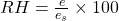, therefore the actual vapour pressure (e) would be 2338 Pa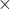0.614 = 1436 Pa. Moreover, if e = 1436 Pa, then the dew-point temperature (Td) would be between 12°C and 13°C.

## Atmospheric Stability

As mentioned in the Lab Overview, atmospheric stability helps determine whether clouds (and precipitation) form or not. The atmosphere could be stable (air parcel remains stationary), unstable (air parcel either rises or sinks), or conditionally unstable.

A key to understand atmospheric stability is the adiabatic process. An adiabatic process is one such that air is heated or cooled due to the expansion or contraction without addition or subtraction of heat. Air will heat or cool at the dry adiabatic lapse rate (10°C/1000m) when the relative humidity is less than 100%. When the relative humidity is 100% (i.e. saturated air), air heats or cools at the moist/saturated adiabatic lapse rate (6°C/1000m on average but varies between 5-9°C/1000m).

In Lab 03, you were introduced to the ideas of a sounding and the environmental lapse rate. The temperature data of the lower atmosphere is typically measured by a weather balloon. Twice a day, meteorologists release a weather balloon where temperatures are recorded at different elevations. The data are transmitted back to the ground where meteorologists can determine the stability.

To determine atmospheric stability, meteorologists would compare the adiabatic lapse rate of an air parcel (either DALR and/or MALR) with the environmental lapse rate (ELR):

1. If ELR > DALR > MALR, there is absolute stability (stable).
2.  If DALR > ELR > MALR, there is conditional instability.
3.  If DALR > MALR > ELR, there is absolute instability (unstable).

For example, here are the data transmitted by a weather balloon:

 Elevation (m) Temp. (°C) 0 25.0 200 22.8 450 20.0 600 18.4 800 16.6 1050 14.4 1200 13.1 1400 12.1 1550 11.3 1800 9.9 2000 8.8

Step 1: Transfer the data onto the Stability Analysis Form:

Step 2: For each layer of the atmosphere, determine the change in elevation (∆ Elev.) and change in temperature (∆ T). For example, layer 1 is between 0 and 200 m. Therefore, ∆ Elev. = 200 m – 0 m = 200 m, and ∆ T = 23.0°C – 22.0°C = 1.0°C.

Step 3: Determine the ELR of all layers of the atmosphere by using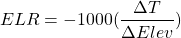Step 4: Determine the stability of all layers of the atmosphere using the values of ELR.

Step 5: Once the Stability Form is completed, you will plot an atmospheric sounding similar to the one you did in Lab 03. This will help us analyze what could happen to an air parcel. The first thing to do is plot the temperature data:

Step 6: For instance, there is an air parcel with temperature (T) of 22°C and dew-point temperature (Td) of 16°C at the surface is forced to rise. Since the air parcel is not saturated with water vapour (i.e. RH < 100%), it will cool at DALR until the dew-point temperature is reached. The elevation at which this occurs is the Lifting Condensation Level (LCL). We can figure this out by using: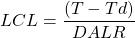In this example,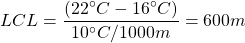Once the air parcel reaches its LCL, the rate of cooling slows to the MALR. If the air parcel rises to 2000 m, we can calculate its temperature by using: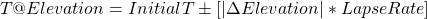In this example,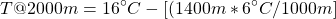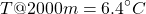If we plot the change in temperature of this air parcel (orange line) and the LCL on the atmospheric sounding, it will look like this:

## Orographic Lifting

An orographic lifting process is where an air parcel is forced to go over a geographic barrier such as a mountain. When the air parcel ascends, air cools adiabatically until it reaches the Lifting Condensation Level (LCL). Consequently, air becomes saturated and water vapour condenses to form clouds. As air descends on the leeward side of the mountain, it warms adiabatically. The following diagram illustrates this concept:

Here is an example of an orographic lifting process. Assume an air parcel at sea-level has temperature of 20°C and dew-point temperature of 15°C. This air parcel is being forced to rise over a 2000m-tall mountain and descend back to sea-level on the other side of the mountain (leeward side).

Step 1: Determine the LCL by usingIn this case,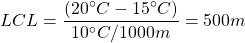Step 2: Determine the temperature of the air parcel when it reaches the summit.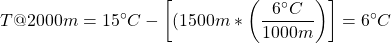Step 3: Determine the temperature of the air parcel when it sinks back to sea-level on the other side of the mountain (lee side).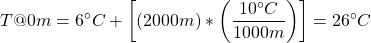# Lab Exercises

In this lab, you will be doing a number of different calculations to help you understand the concepts of atmospheric moisture and stability. A calculator is required, and you will also be plotting a graph – your instructor will decide if you will use a graph paper or a spreadsheet. Please make sure to show all your calculations (to maximize partial credits) and not just the answers.

EX1: Moisture Variables

1. Complete the following table (in Worksheets). Give answers for RH and Td to one decimal place, e to the nearest integer. (The tables required for this exercise are in the Supporting Material section.) Show your calculations separately.
Dry-bulb temperature

(T)

Wet-bulb temperature

(Tw)

Wet-bulb depression

(T-Tw)

Relative humidity

(RH)

Saturation vapour pressure

(es)

Actual vapour pressure

(e)

Dew point temperature

(Td)

a) 13°C 11°C _______ _______ _______ _______ _______
b) 15°C _______ 6°C _______ _______ _______ _______
c) 28°C _______ _______ 70.1% _______ _______ _______
d) 20°C N/A N/A _______ _______ _______ 13.0°C
e) 5°C _______ _______ _______ _______ 705 Pa _______
f) ________ _______ _______ _______ 3779 Pa 2267 Pa _______
g) 24°C _______ _______ _______ _______ _______ 24.0°C

EX2: Atmospheric Moisture

1. On a cold snowy day, the outside air temperature is 0°C and the relative humidity is 100%. The air is taken into the house and heated to 21°C. What is the relative humidity inside the house?
2. Dew is often formed on grass early in the morning but not at other times of the day. Explain why this is.
3. Go to this website and keep track of the relative humidity over the course of 3-4 days. Do you feel more comfortable when the RH is high, or low? Can you explain what are the major factors that affect RH?
4. Search online to acquire the company names and model numbers of at least two different instruments (other than the sling psychrometre) for sensing humidity.

EX3: Atmospheric Stability

1. A meteorological balloon is sent aloft and temperature data is obtained (see the attached atmospheric stability analysis form). Plot the vertical temperature profile on a metric graph paper. Use a horizontal scale of 1 cm = 2°C and a vertical scale of 1 cm = 200 m. Label this line “Environmental Air”.
2. Complete the atmospheric stability analysis form provided (in Worksheets). Give your answers for ELR to one decimal place.
3. An air parcel of 24°C and dew point temperature of 16°C is the surface. Assuming that the air parcel is forced to rise, plot a line showing the hypothetical change in temperature of this rising parcel on the same graph. Label this line “Air Parcel”. On the line indicate where the rising parcel of air cools at the DALR and where it cools at the MALR, assuming a dry adiabatic lapse rate of 10°C / km and a moist adiabatic lapse rate of 6°C / km.
4. What is the lifting condensation level (LCL)? Use a horizontal line and mark the LCL on the graph.

Submit the Stability Analysis Form and your graph.

EX4: Orographic Process

Air blowing off the Pacific has a temperature of 15°C and a dew-point temperature of 10°C at Vancouver (sea level). This air is forced to rise over the Coast Range on its way to Calgary.

1. What is the LCL?
2. What is the temperature of the air parcel at the top of the Coast Range (elevation = 2200 m)?
3. What is the temperature of the air parcel in Calgary (elevation = 1200m)?

Reflection Questions

1. In EX4 (Orographic Process), if we changed the temperature of the air parcel in Vancouver to 30°C and dew-point temperature to 7°C, what would be the temperature of the air parcel when it reaches Calgary? Briefly explain what happen.
2. Take a picture of something around you, whether that is at home or somewhere outside, that illustrates one of the processes of phase change. Include one or two sentences to describe what that example is. Do not use a scenario that has already been discussed in class.
3. Draw a line on the picture below (Figure 7.9) indicating where the Lifting Condensation Level (LCL) is. Briefly explain your reasoning.

# Worksheets

EX1 Worksheet

Atmospheric Stability Analysis Form

# Supporting Material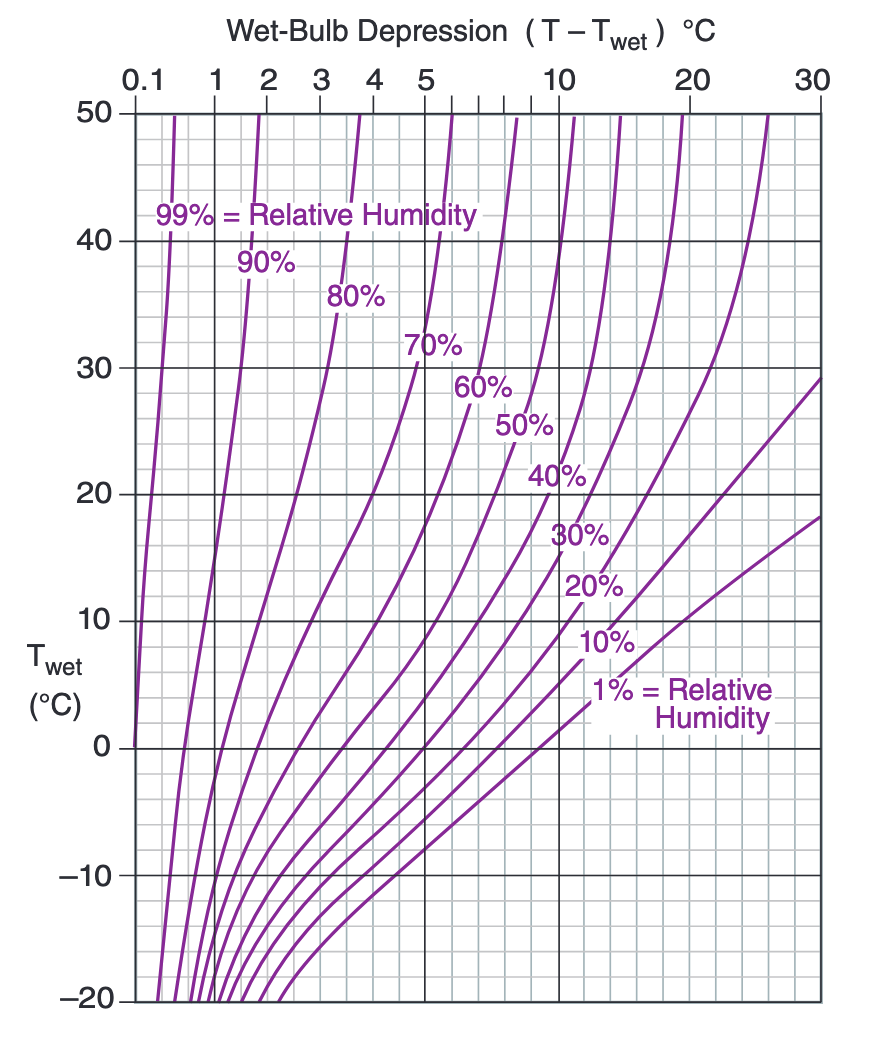Figure 7.10 Psychrometric graph, to find relative humidity from wet and dry-bulb temperatures. Figure courtesy of R. Stull

### References

Geography Department, Langara College, 2017, Geography 1180 Lab Manual

Steward, M. Orographic Effect. https://www.flickr.com/photos/megstewart/8644087724/. Accessed August 13, 2020.

Stull, R., 2017: Practical Meteorology: An Algebra-based Survey of Atmospheric Science -version 1.02b.  Univ. of British Columbia.